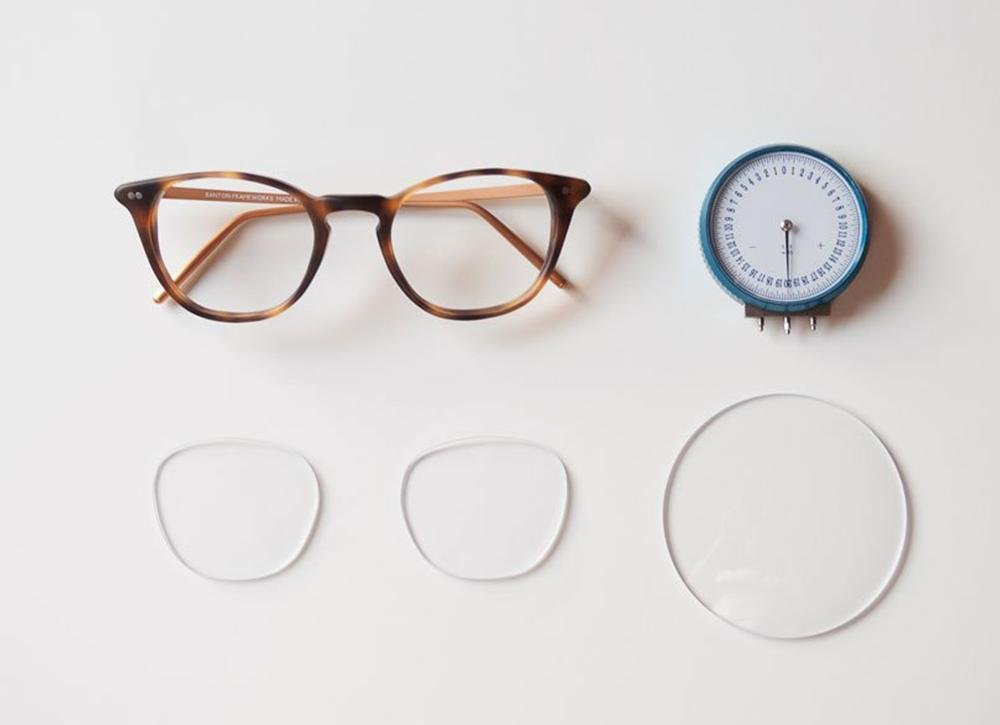0

Go to the shop
Close

## Do you really understand the lenses of glasses?### The refractive index

Refraction law is discovered by Dutch mathematician Snell.It is the law that determines the direction of refracted light in the phenomenon of light refraction. When the light from the first medium (refractive index N1) into the second medium (refractive index N2), part of the light from the first medium into the second medium at the smooth interface will be refracted. The index of refraction is the ratio of the speed at which light travels in a vacuum to the speed at which light travels in the medium. For glasses lenses, the higher the refractive index, the thinner the lens, that is, the lens with the same center thickness and the same degree, the lens with the higher refractive index has thinner edges than the lens with the lower refractive index of the same material.

### How do you identify the refractive index?

In reality, this problem is often a problem for optical shop practitioners, sometimes they worry about whether the manufacturer's lenses match the nominal value of the refractive index. We need a prop, and that's the camber. Because it can be used to measure the "strength" of the lens. The luminosity of the lens can be calculated by subtracting the curvature of the inner and outer surfaces of the lens. The curvature difference of a lens of the same luminosity is inversely proportional to the refractive index, that is, a lens with a higher refractive index only needs a smaller curvature difference to achieve the same luminosity.

\$42.00

\$4.99 \$42.00

### What is the processing technology to make them thin?

For myopic lenses, the larger the diameter, the thicker the edge. Therefore, for customers with high myopia, we will recommend frames with smaller ring diameters, so that the edges of the processed lenses will be thinner. The edges of farsightedness lenses are already very thin (convex lenses are thick in the middle and thin on the sides), and the key determinant of lens thickness is the center thickness. In addition to the refractive index, which affects the thickness of the farsightedness lens, the influence of diameter is more important. Therefore, for patients with farsightedness, the most effective way to make lenses lighter and thinner is to choose a smaller frame and then customize a pair of lenses with the right diameter.

### Parameters of lens material selection

Abbe number

Since the refractive index of the lens material varies for the light of different wavelengths, and white light is made up of various colors of light of different wavelengths, the dispersion phenomenon occurs when the lens refracts white light. The dispersion coefficient is an important index to identify the sharpness of lens imaging, which is usually indicated by Abbe number (Abbe number = dispersion coefficient). The larger the Abbe number, the smaller the dispersion, and vice versa, the smaller the Abbe number, the larger the dispersion, and the worse the resolution of the image.

Impact resistance

Humans have been using glass lenses for about 300 years, and it was not until 1947 that the first resin lenses made of CR39 were produced. A big reason for using resin lenses is their safety, i.e. impact resistance. Only lenses that pass the Drop Ball Test are considered safe. Combining the above two parameters, we can compare different lenses.

Tags : Eyeglasses
categories : The Blog